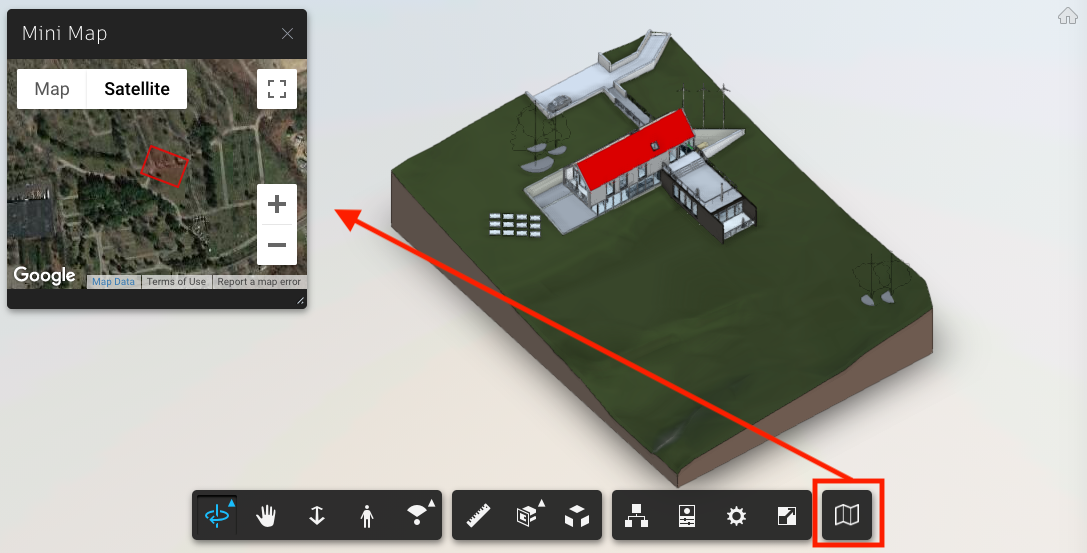March 25, 2019

# Mini-map with Geolocation extensionThis cool extension is available since version 6.4 (see this post) and brings a few functions to handle conversions between Viewer XYZ and LL84 Lat Long coordinate system. For this post, let's implement a mini-map with the current model position and bounding box. Let's call it MiniMapExtension and use the skeleton described here.

See it live here. Note you'll need a model with location.

For the code, first, we need a map with API, let's use Maps JavaScript. You'll need a developer key to include into your HTML file, like this:

``<script async defer src="https://maps.googleapis.com/maps/api/js?key=YOU_KEY_HERE" type="text/javascript"></script>``

The following code shows how to initialize a map. Note how the center is 0/0, we'll update it later.

``````// initialize the map
if (_this.map == null) {
zoom: 16,
center: { lat: 0, lng: 0 },
mapTypeId: 'satellite',
rotateControl: false,
streetViewControl: false,
tilt: 0
});
// draw model boundoung box & center
var bb = _this.viewer.model.getBoundingBox();
_this.drawBoundingBox(bb.min, bb.max);
_this.cameraChanged(_this.viewer.autocam); // first run (center of the model)
}``````

Viewer gives us the bounding box (min/max) information, used above. The following code uses it to create a polygon and draw it using Maps Shapes API.

``````MiniMapExtension.prototype.drawBoundingBox = function (min, max) {
// basic check...
if (this.map == null) return;
if (this.geoExtension == null) return;

// prepare a polygon with the bounding box information
var polygon = [];
polygon.push({ x: min.x, y: min.y });
polygon.push({ x: min.x, y: max.y });
polygon.push({ x: max.x, y: max.y });
polygon.push({ x: max.x, y: min.y });

this.drawPolygon(polygon);
}

MiniMapExtension.prototype.drawPolygon = function (polygon) {
// basic check...
var _this = this;
if (_this.map == null) return;
if (_this.geoExtension == null) return;

// prepare the polygon coordinate to draw it
var coords = [];
polygon.forEach(function (point) {
var geoLoc = _this.geoExtension.lmvToLonLat(point);
coords.push({ lat: geoLoc.y, lng: geoLoc.x });
});
var polyOptions = {
path: coords,
strokeColor: '#FF0000',
strokeOpacity: 0.8,
strokeWeight: 2,
fillColor: '#FF0000',
fillOpacity: 0.1,
};
polygon.setMap(_this.map);
}``````

The above code uses lmvToLonLat() function, which essentially converts from Viewer coordinates to LL84. The opposite can be done with lonLatToLmv(), but will leave that for a future experiment. A mini-map needs to sync with the model, right? So let's use the viewer.autocam.center point to position the map. To make it interactive, we need to addEventListener to CAMERA_CHANGED event.

``````MiniMapExtension.prototype.cameraChanged = function (camera) {
// basic check...
if (this.map == null) return;
if (this.geoExtension == null) return;

// adjust the center of the map
var geoLoc = this.geoExtension.lmvToLonLat(camera.center);
this.map.setCenter({ lat: geoLoc.y, lng: geoLoc.x });
}``````

Last, you need a CSS to define the button and maps and to make Viewer load it.

And get the entire extension code here.